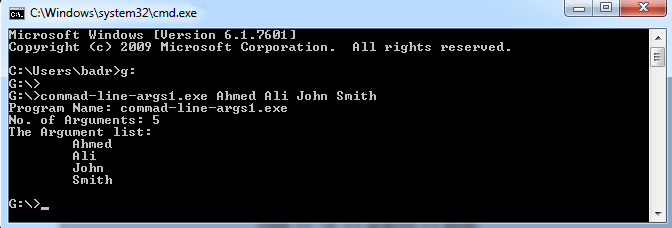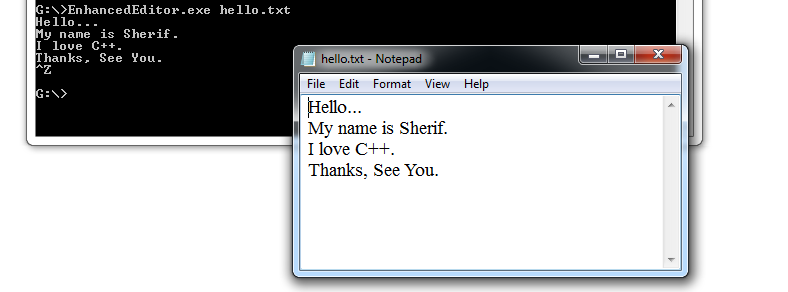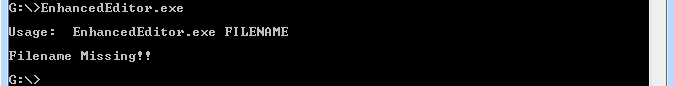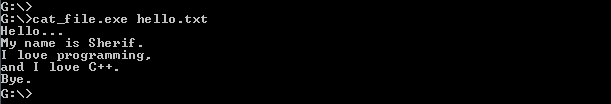# Learn Using Command-Line Arguments in C++

0
3737Another way to provide inputs to programs is through Command-Line Arguments. If you are a UNIX/Linux guy, you should be familiar with arguments provided to commands/scripts at runtime. In this article, we will discuss how to use command-line arguments in C++. Wish you nice reading.

argc and argv[]
In all the programs we have written so far in this series, you may notice that the main() function came with no arguments. We have defined main() functions that return void (nothing), and others that return int (integer), but all of them share one common thing: no arguments. Actually, that is not a rule. The main() function could accept two arguments:

argc – (argument count) an integer variable that stores the number of command-line arguments passed to the program.

argv[] – (argument values) an array of strings that contains the provided command-line arguments. The first element in this array argv is the name of the program. The remaining elements from argv to argv[n] represents arguments from the first to the nth.

Example
The following program prints the program name, number of provided arguments, and the list of arguments.

```#include <iostream>
using namespace std;
int main(int argc, char** argv)
{
cout << "Program Name: " << argv << endl
<< "No. of Arguments: " << argc << endl
<< "The Argument list:\n";
for(int i=1;i<argc;i++)
cout << '\t' << argv[i] << endl;
return 0;
}
```

Let’s run it and see what we get.Enhanced Text Editor Example
In the previous article, we have implemented a simple text editor. That editor starts by prompting the user to enter a filename, and then prompts for text that will be the file contents. That is not friendly enough. The following code presents a better implementation:

```/*	Enhanced Text Editor 	*/
#include <iostream>
#include <fstream>
using namespace std;
int main(int argc, char *argv[])
{
if(argc!=2)
{
cout << "\nUsage:\t" << argv << " FILENAME\n";
cout << "\nFilename Missing!!\n";
return 1;
}
string filename = argv;
int MAX = 1000;
char text[MAX];
ofstream outfile(filename.c_str());
cin.get(text,MAX,'~');
outfile << text;
outfile.close();
return 0;
}
```

Let’s try our new Text Editor.If you forget to provide a filename (as an argument in the command line), the program prints a usage message, and exits.For the program to work correctly, it should check if the user has provided an argument (filename). The user should provide one (and only one argument). In this case the number of arguments argc should be 2.

The condition:

`if(argc!=2)`

will be true if the user has provided incorrect number of arguments (no arguments, or more than one). If so, the program prints a usage message, and exits.

Cat Command Emulator Example
The following code emulates the function of the UNIX/Linux cat command. For non-UNIX guys, the cat command reads the contents of a file and prints it to the standard output.

```#include <iostream>
#include <string.h>
#include <fstream>
#include <stdlib.h>
using namespace std;
int main(int argc, char** argv)
{
string filename;
int MAX=1000;
char str[MAX];
if(argc !=2)
{
cout << "\nUsage:\t" << argv << " FILENAME\n";
cout << "\nFilename Missing!!\n";
exit(1);
}
filename = argv;
ifstream FILE(filename.c_str());
if(!FILE)
{
cout<<"Error in opening file..!!";
exit(2);
}
while(FILE.eof()==0)
{
FILE.getline(str,MAX);
cout << str << endl;
}
cout<<"\n";
FILE.close();
return 0;
}
```

When compiled and executed, the program should read a file and print its contents.Formidable!!!

Summary
Providing command-line arguments at programs’ execution is a way to provide inputs.
The main() function could accept two arguments: the number of command-line arguments argc, and the list of arguments provided argv[].

I hope you find this useful. See you in the next article.